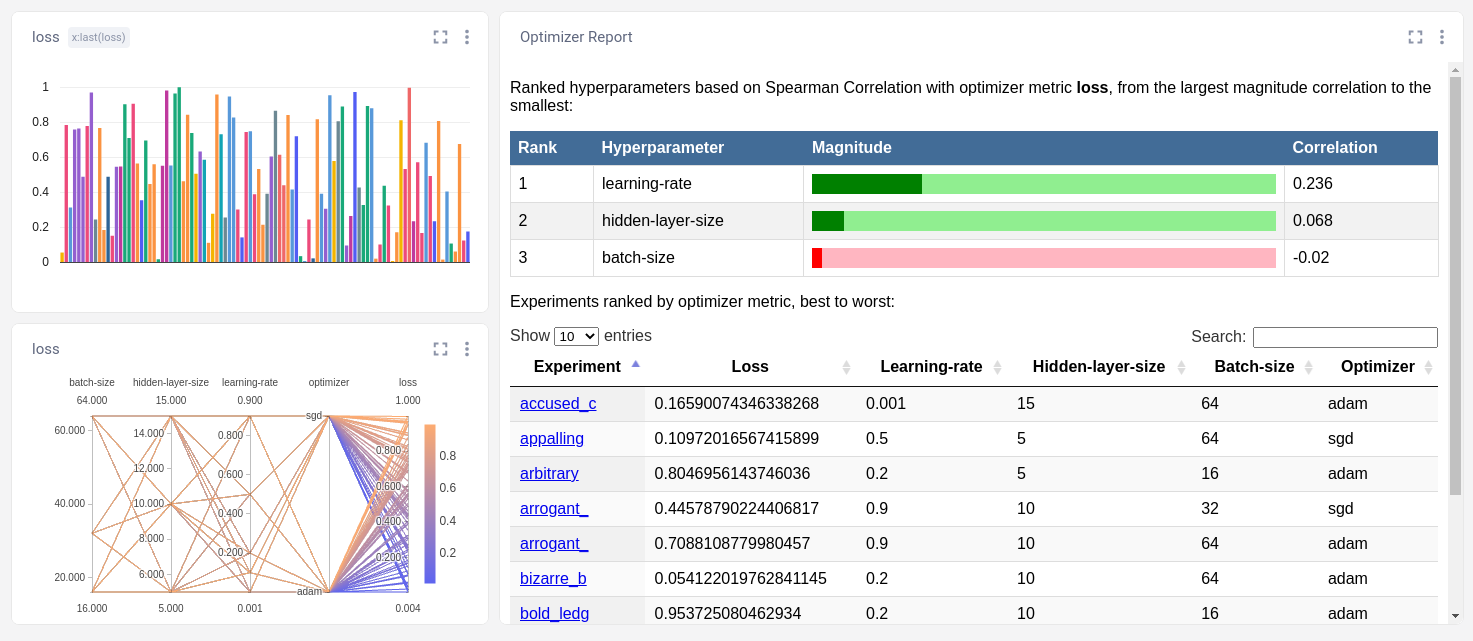# Third-Party Optimizers

Although Comet provides a powerful Hyperparameter Optimizer designed for experiment management and different search algorithms (including random, grid, and bayes), you can also use your own, or any third-party optimizer/search system.

When you create an Experiment with Comet's Optimizer, you also get the support of a number of related tools, including the Optimizer Report Panel and Parallel Coordinate Report:However, you can also use these related tools with your own optimizer. You need only make sure that all of the hyperparameters are logged, and log these additional "other" items:

```import json, os
# parameters is a dict of hyperparameter names/values
# experiment is a Comet experiment
experiment.log_other("optimizer_metric", "loss") # name of metric
experiment.log_other("optimizer_metric_value", 0.7)
experiment.log_other("optimizer_version", "diy-1.0") # your own info
experiment.log_other("optimizer_process", os.getpid()) # if you wish
experiment.log_other("optimizer_count", 23) # the combination count so far
experiment.log_other("optimizer_objective", objective) # "minimum" or "maximum"
experiment.log_other("optimizer_parameters", json.dumps(parameters)) # string of parameters
experiment.log_other("optimizer_name", "optional-name") # string
```

Below you will find a complete, but simple, Do It Yourself (DIY) example search algorithm. Note that there are many limitations to this, including:

1. when selecting a combination randomly, all combinations are in memory and could crash your computer if there are too many
2. if a training example doesn't complete (e.g. it crashes) then there is no mechanism to try the combination again
3. only runs one trial for each combination
4. can't be used in a distributed manner (need a centralized server to provide combinations)
5. only does random and grid search, not a sophisticated Bayes search

You can substitute a proper hyperparameter search for this simple version. Of course, Comet's Optimizer solves all of the above issues.

```# A DIY Hyperparameter search

from comet_ml import Experiment

import itertools
import json
import random
import os

def get_parameters(params, shuffle=True):
"""
Return a dictionary of parameter settings given
lists of possible values for each.

Args:
params: dict of lists of possible values
shuffle: bool; if True, shuffle the value orders

Returns a dict of key/value for each combination.
"""
if shuffle:
combinations = []
for values in itertools.product(*params.values()):
combinations.append(dict(zip(params, values)))
random.shuffle(combinations)
for combo in combinations:
yield combo
else:
for values in itertools.product(*params.values()):
yield dict(zip(params, values))

hyperparameters = {
"learning-rate": [0.001, 0.1, 0.2, 0.5, 0.9],
"batch-size": [16, 32, 64],
"hidden-layer-size": [5, 10, 15],
}

def train(**hyperparams):
# a dummy training function that returns loss
return random.random()

count = 0
metric_name = "loss"
objective = "minimize"
for parameters in get_parameters(hyperparameters):
count += 1
experiment = Experiment(project_name="diy-search")
experiment.log_parameters(parameters)
metric_value = train(**parameters)
experiment.log_metric(metric_name, metric_value, step=0)

experiment.log_other("optimizer_metric", metric_name)
experiment.log_other("optimizer_metric_value", metric_value)
experiment.log_other("optimizer_version", "diy-1.0")
experiment.log_other("optimizer_process", os.getpid())
experiment.log_other("optimizer_count", count)
experiment.log_other("optimizer_objective", objective)
experiment.log_other("optimizer_parameters", json.dumps(parameters))
experiment.log_other("optimizer_name", "diy-optimizer-001")
```